## 纯Javascript的简单弱智提问

cy904159293 发布于 2013/07/24 09:04

1，关于Js数组的，这个申明数组为什么是可以的？

function imgl(x) { //获取数组的记录数
this.length = x;
}
//声明数组并给数组元素赋值，也就是把图片的路径保存起来
imgs = new imgl(2);
imgs = "14.jpeg";
imgs = "mm1.jpg";

2，一段顺序执行过程中，注释部分如果用的话，就起不了作用

// i = (i == 1) ? 0 : i++;
if (i == 1) { i = 0;}
else { i++; }

var i = 1;
i = (i ==1 ) ? 0 : i++;
alert(i); // 0

i = 0;
i = (i ==1) ? 0 : i++;
alert(i); // 还是0，这就是纳闷之处（ps:外加括号一样的）

0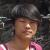c

0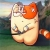1.imgs是一个对象。imgs其实相当于给这个对象加了一个属性，与imgs["name"] = "coco"是一样的。这并不是一个真正的数组，数组应有的操作如push这些是不能使用的。

..第二个没看懂

0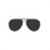2. i =( (i == 1) ? 0: i++);
c

0

1.脑残之极，如果参数带入的是字符串呢。

还要去判断。还不如直接就用一个对象去处理，要获取对象的长度难道很困难吗。

2.i =( (i == 1) ? 0: i++);

else { i++; } 最后结果为0。

0
c

#### 引用来自“柔软胖子”的答案

1.imgs是一个对象。imgs其实相当于给这个对象加了一个属性，与imgs["name"] = "coco"是一样的。这并不是一个真正的数组，数组应有的操作如push这些是不能使用的。

..第二个没看懂

<script>
function myArray(length) {
this.length = length;
}
var arr = new myArray(2);
arr["first"] = "First";
arr["second"] = "Second";
arr = "0";
alert(arr);
</script>

if (i == 1) {i = 0} //是1变回0
else {i++} //这样 i 就会无限 0 和 1 之间变值了，

0
c

#### 引用来自“云中漫步”的答案

1.脑残之极，如果参数带入的是字符串呢。

还要去判断。还不如自己就用一个对象去处理，要获取对象的长度难道很困难吗。

2.i =( (i == 1) ? 0: i++);

else { i++; } 最后结果为0。

1，呵呵，我是看到有人这样注释的，那我就纳闷了，Array还有这样用的，那这个对象的长度获取咋实现呐，新手新手 - -

2，流程什么的我晓得了，我纳闷如下：

var i = 1;
i = (i ==1 ) ? 0 : i++;
alert(i); // 0

i = 0;
i = (i ==1) ? 0 : i++;
alert(i); // 还是0，这就是纳闷之处（ps:外加括号一样的）

c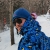0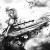imgs=[]就够了，简洁又方便

i =i++这一句在js中等同于i=i，因为最后赋值给i的结果是在自增之前的结果，覆盖了自增后的值

c

0c